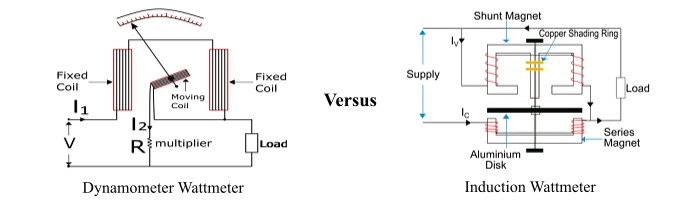# Difference between Dynamometer and Induction Type Wattmeter

A wattmeter is an electrical measuring instrument that measures active (or real) power in an electric circuit. It measures the value of electric power in watts, kW, MW, etc.

Based on the principle and construction, the wattmeters are classified into two major types as −

• Dynamometer type wattmeter
• Induction type wattmeter

The primary function of both dynamometer and induction type wattmeter is the same, i.e. measurement of active power in the circuit. However, these two types of wattmeters are different from each other in many aspects.In this article, you will learn all the differences between dynamometer wattmeter and induction wattmeter. But, before that, let's get some basic information about the dynamometer type and the induction type wattmeters so that it becomes easier to understand how they are different from each other.

## What is a Dynamometer Type Wattmeter?

A dynamometer type wattmeter is an instrument that is universally used for the measurement of DC as well as AC electric power. It is also called Electrodynamometer wattmeter.

It works on the principle that a mechanical force acts between two current carrying conductors.

An electrodynamometer wattmeter has a fixed coil divided into two parts and is connected in series with the load and caries the load current. The moving coil is connected across the load through a series multiplier resistance and carries a current proportional to the load voltage. The fixed coil is called as current coil and the moving coil is called as potential coil (pressure coil). The controlling torque is provided by two spiral springs. Air friction damping is provided in electrodynamometer wattmeter. A pointer is attached with the moving coil which deflects to on a calibrated scale to show the reading of power.

The major advantage of a dynamometer type wattmeter is that it can measure both AC and DC power.

## What is an Induction Type Wattmeter?

An induction type wattmeter is a measuring device that can measure electric power in AC circuits only. It works on the principle of electromagnetic induction.

An induction wattmeter consists of two laminated electromagnets viz. Shunt Magnet and Series Magnet. The shunt magnet is connected across the supply and carries a current proportional to the supply voltage. The coil of shunt magnet is made highly inductive so that the current in it lags the supply voltage by 90°. The series magnet is connected in series with the supply and carries the load current. The coil of series magnet is made highly non-inductive. A thin disc (made up of aluminium) mounted on a spindle is placed between the two magnets so that it cuts the flux of the two magnets.

When the wattmeter is connected in an AC circuit, a current flows through the coil of the shunt magnet that is proportional to the supply voltage and the series magnet carries the load current. The fluxes produced by the two magnets induce eddy currents in the aluminium disc by the action of electromagnetic induction. Due to the interaction between the fluxes and eddy currents, a deflecting torque is produced on the disc, causing the disc to move and hence, the pointer connected to the disc moves over the scale and shows the value of power.

## Difference between Dynamometer Wattmeter and Induction Wattmeter

The function of both wattmeters is the same, i.e. measuring of active power in an electric circuit. However, there are several differences between dynamometer type wattmeter and induction type wattmeter that are highlighted in the following table −

Basis of Difference Dynamometer Type Wattmeter Induction Type Wattmeter
Description An electrical measuring device that operates on the principle of electrodynamometer and can measure real power in an AC circuit as well DC circuit is called a dynamometer type wattmeter. An electrical measuring instrument that works on the principle of electromagnetic induction and can measure AC power only is called induction type wattmeter.
Operating principle The operation of dynamometer type wattmeter is based on the fact that a mechanical force acts between two current carrying conductors. Induction wattmeter operates on the principle of electromagnetic induction, i.e. a changing magnetic field can induce an emf in a conductor.
Circuit The dynamometer type wattmeter can be used in both AC and DC circuits to measure the electrical power. The induction wattmeter can be used in AC circuits only.
Accuracy Dynamometer wattmeter is more accurate. Induction type wattmeter is comparatively less accurate.
Parts Dynamometer wattmeter consists of two coils namely, current coil and pressure coil, scale and pointer. The main parts of induction wattmeter are: shunt electromagnet, series electromagnet, aluminium disc, scale and pointer.
Power consumption Dynamometer wattmeter consumes less power. Induction wattmeter consumes comparatively high power.
Moving coils In dynamometer wattmeter, the pressure coil acts moving coil. Induction wattmeter has no moving coils, rather it has an aluminium disc as moving part.
Effect of changing frequency & voltage The operation of dynamometer wattmeter is not affected by fluctuating voltage and frequency. The operation of induction wattmeter is greatly affected by fluctuations in voltage and frequency.
Weight of moving system Dynamometer wattmeter has relatively less heavy moving system. The moving system of induction wattmeter is heavier than dynamometer wattmeter.
Damping Dynamometer type wattmeter uses air friction damping. Induction wattmeter uses eddy current damping.

## Conclusion

The major difference between a dynamometer type wattmeter and an induction type wattmeter is that a dynamometer wattmeter can measure both AC and DC power, whereas an induction wattmeter can measure only AC power.

Updated on: 19-Aug-2022

3K+ Views